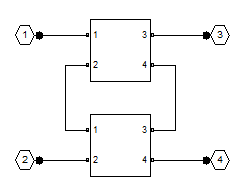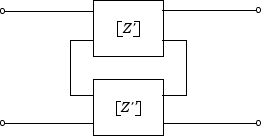# rfckt.series

Series connected network

## Description

Use the `series` class to represent networks of linear RF objects connected in series that are characterized by the components that make up the network. The following figure shows a pair of networks in a series configuration.## Creation

### Syntax

``h = rfckt.series``
``h = rfckt.series('Ckts',value)``

### Description

````h = rfckt.series` returns a series connected network object whose properties all have their default values.```

example

````h = rfckt.series('Ckts',value)` returns a series connected network with elements specified in the name-value pair property `Ckts`.```

## Properties

expand all

Computed S-parameters, noise figure, OIP3, and group delay values, specified as rfdata.data object. `Analyzed Result` is a read-only property. For more information, see Algorithms.

Data Types: `function_handle`

Circuit objects in network, specified as a cell array of object handles. All circuits must be 2-port. By default, this property is empty.

Data Types: `char`

Object name, specified as a `1-by-N` character array. `Name` is a read-only property.

Data Types: `char`

Number of ports, specified as a positive integer. `nport` is a read-only property. The default value is `2`.

Data Types: `double`

## Object Functions

 `analyze` Analyze RFCKT object in frequency domain `calculate` Calculate specified parameters for rfckt objects or rfdata objects `circle` Draw circles on Smith Chart `extract` Extract specified network parameters from rfckt object or data object `listformat` List valid formats for specified circuit object parameter `listparam` List valid parameters for specified circuit object `loglog` Plot specified circuit object parameters using log-log scale `plot` Plot circuit object parameters on X-Y plane `plotyy` Plot parameters of RF circuit or RF data on X-Y plane with two Y-axes `getop` Display operating conditions `polar` Plot specified object parameters on polar coordinates `semilogx` Plot RF circuit object parameters using log scale for x-axis `semilogy` Plot RF circuit object parameters using log scale for y-axis `smith` Plot circuit object parameters on Smith chart `write` Write RF data from circuit or data object to file `getz0` Calculate characteristic impedance of RFCKT transmission line object `read` Read RF data from file to new or existing circuit or data object `restore` Restore data to original frequencies `getop` Display operating conditions `groupdelay` Group delay of S-parameter object or RF filter object or RF Toolbox circuit object

## Examples

collapse all

Create a series connected RF network object using `rfckt.series`

```tx1 = rfckt.txline; tx2 = rfckt.txline; ser = rfckt.series('Ckts',{tx1,tx2})```
```ser = rfckt.series with properties: Ckts: {[1x1 rfckt.txline] [1x1 rfckt.txline]} nPort: 2 AnalyzedResult: [] Name: 'Series Connected Network' ```

## Algorithms

The `analyze` method computes the S-parameters of the `AnalyzedResult` property using the data stored in the `Ckts` property as follows:

1. The `analyze` method first calculates the impedance matrix of the series connected network. It starts by converting each component network's parameters to an impedance matrix. The following figure shows a series connected network consisting of two 2-port networks, each represented by its impedance matrix,where

`$\begin{array}{l}\left[{Z}^{\prime }\right]=\left[\begin{array}{cc}{Z}_{11}{}^{\prime }& {Z}_{12}{}^{\prime }\\ {Z}_{21}{}^{\prime }& {Z}_{22}{}^{\prime }\end{array}\right]\\ \left[{Z}^{″}\right]=\left[\begin{array}{cc}{Z}_{11}{}^{\prime \text{​}\prime }& {Z}_{12}{}^{\prime \text{​}\prime }\\ {Z}_{21}{}^{\prime \text{​}\prime }& {Z}_{22}{}^{\prime \text{​}\prime }\end{array}\right]\end{array}$`

2. The `analyze` method then calculates the impedance matrix for the series network by calculating the sum of the individual impedances. The following equation illustrates the calculations for two 2-port circuits.

`$\left[Z\right]=\left[{Z}^{\prime }\right]+\left[{Z}^{″}\right]=\left[\begin{array}{cc}{Z}_{11}{}^{\prime }+{Z}_{11}{}^{\prime \text{​}\prime }& {Z}_{12}{}^{\prime }+{Z}_{12}{}^{\prime \text{​}\prime }\\ {Z}_{21}{}^{\prime }+{Z}_{21}{}^{\prime \text{​}\prime }& {Z}_{22}{}^{\prime }+{Z}_{22}{}^{\prime \text{​}\prime }\end{array}\right]$`

3. Finally, `analyze` converts the impedance matrix of the series network to S-parameters at the frequencies specified in the analyze input argument `freq`.

 Ludwig, Reinhold and Pavel Bretchko, RF Circuit Design: Theory and Applications, Prentice-Hall, 2000.